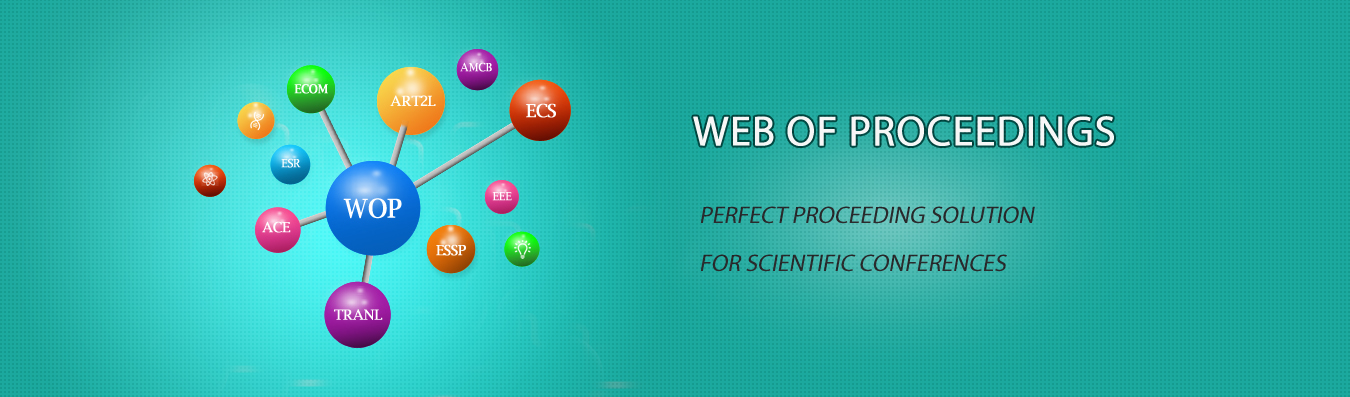The best way to conference proceedings by Francis Academic Press### Iterative Solution of the Three-Body Problem and System Simulation

DOI: 10.25236/icited.2021.099

Qifeng He

Qifeng He

#### Abstract

The three-body problem is a fundamental model in astrophysics that studies the law-of-motion problem of three objects that are considered as masses under the gravitational influence. This paper utilizes classical mechanical equations and computer's advantages in terms of numerical operations to iteratively solve the three-body prob¬lem and determine its bound state. In this experiment, the three objects are analyzed by force; equations are listed using Newton's second law and law of universal gravitation; and the MATLAB program is written ac¬cording to the equations for iterative solution, which calculates the position and velocity of the three objects at a specific time under different circumstances. In addition, in this paper, the Gui design and animation of MATLAB are utilized to investigate and display the problem. A simple and friendly interface is designed, the position and velocity of the objects are also shown on the axes in real time through the erasure animation, which is an attempt to perform astrophysical experiments on the computer.

#### Keywords

Three-body problem-Bound state-iterative method-System Simulation